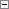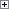Returns a pseudo-random number.

Number := RANDOM(MaxNumber)

Parameters

MaxNumber

Type: Integer

The largest acceptable number. In effect, you are setting a range from one (1) to the number that you specify with the MaxNumber parameter.Property Value/Return Value

Type: Integer

A pseudo-random number between one (1) and the number that you entered for MaxNumber.Remarks

If MaxNumber is negative it acts as a positive.

If MaxNumber is zero, this function always returns 1.

A number is always chosen from the same set of numbers. Use RANDOMIZE Function (Integer) to generate a new set of numbers.Example

This example shows how to generate a pseudo-random number. This example requires that you create the following variables and text constant in the C/AL Globals window. The value of the variable Number2 is positive though the value of MaxNumber is negative and the value of the variable Number3 is always 1 because MaxNumber is 0.

Variable name DataType

x

Integer

y

Integer

z

Integer

Number1

Integer

Number2

Integer

Number3

Integer

Text constant name ENU Value

Text000

Number1 = %1, Number2 = %2, Number3 = %3Copy Code
x := 100; // x is assigned a positive value.
y := -100; // y is assigned a negative value.
z := 0; // z is assigned zero.
Number1 := RANDOM(x);
Number2 := RANDOM(y);
Number3 := RANDOM(z);
MESSAGE(Text000, Number1, Number2, Number3);